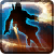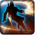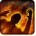# Flame SweepConditions

Used by

## Related effects, buffs and debuffs

Please click on an effect below to view its details.

• [0s] [not displayed]Effect #1

 Slot: EffectOther Duration: 0s Tick rate: does not tick # occurrences: 0 Target overrides: [target override] Aoe Sphere
• On Apply

Perform the following actions:

• Play appearance epp . bounty_hunter . flame_sweep . flame_sweep_cast
• On Apply
• [target override]

Only when the following conditions are met:

• <
• If PRIMARY TARGET is alive
AND
• If target is an enemy
>

Perform the following actions:

• Add effect #2 to TARGET from TARGET
• [hidden] [0s]Effect #2

 Slot: Debuff Duration: 0s Tick rate: does not tick # occurrences: 0 Tags: tag.​abl.​generic.​fire_damage, tag.​abl.​utility.​is_aoe, tag.​abl.​bounty_hunter.​aoe_flame_damage
• On Apply

Only when the following conditions are met:

• Can only by called by other effects

Perform the following actions:

• Invoke Projectile
- Determine Hit = (bool) false
- Timed Projectile = (bool) false
- Hit Checked = (bool) false
- Is Special Ability = (bool) false
- Ignore Dual Wield Modifier = (bool) false
- Slot = (int) 5
- Spell Type = (int) 1
- Travel Speed = (float) 1
- Travel Time = (time) 0s
• When projectile completed travel and hit target

Perform the following actions:

• Play appearance epp . bounty_hunter . flame_sweep . flame_sweep_burn
• Ballistic Impulse, centered at caster, never knockdown, momentum = 2, 0° pitch, 0° yaw
• Add effect #3 to TARGET from TARGET
• Add effect #4 to TARGET from TARGET
• [5s]Burning
Periodic elemental damage.

 Slot: Debuff Duration: 5s Tick rate: 1s # occurrences: 1 Tags: tag.​abl.​debuff.​damage_over_time, tag.​abl.​debuff.​burn Conditions: Can only by called by other effects
• On Apply

Perform the following actions:

• Play appearance epp . npc . ability . tech . flamethrower . flamethrower_target_burn, dependent on calling effect
• When effect ticks

Only when the following conditions are met:

• If difficulty is set to 16-man

Perform the following actions:

• Spell Damage
- Unknown (609) = (bool) false
- Damage Type = (int) 3
- Slot = (int) 1
- Spell Type = (int) 2
- Level Cap = (int) 0
- Amount Max = (float) 0
- Amount Min = (float) 0
- Amount Percent = (float) 0
- Threat Percent = (float) 0
- Standard Health Percent Max = (float) 0.04
- Standard Health Percent Min = (float) 0.04
- Amount Modifier Percent = (float) 0
- Coefficient = (float) 0
- Health Steal Percentage = (float) 0
• When effect ticks

Only when the following conditions are met:

• If difficulty is set to 8-man SM

Perform the following actions:

• Spell Damage
- Unknown (609) = (bool) false
- Damage Type = (int) 3
- Slot = (int) 1
- Spell Type = (int) 2
- Level Cap = (int) 0
- Amount Max = (float) 0
- Amount Min = (float) 0
- Amount Percent = (float) 0
- Threat Percent = (float) 0
- Standard Health Percent Max = (float) 0.015
- Standard Health Percent Min = (float) 0.015
- Amount Modifier Percent = (float) 0
- Coefficient = (float) 0
- Health Steal Percentage = (float) 0
• When effect ticks

Only when the following conditions are met:

• If difficulty is set to 8-man HM

Perform the following actions:

• Spell Damage
- Unknown (609) = (bool) false
- Damage Type = (int) 3
- Slot = (int) 1
- Spell Type = (int) 2
- Level Cap = (int) 0
- Amount Max = (float) 0
- Amount Min = (float) 0
- Amount Percent = (float) 0
- Threat Percent = (float) 0
- Standard Health Percent Max = (float) 0.04
- Standard Health Percent Min = (float) 0.04
- Amount Modifier Percent = (float) 0
- Coefficient = (float) 0
- Health Steal Percentage = (float) 0
• [0s] [not displayed]Effect #4

 Slot: Debuff Duration: 0s Tick rate: does not tick # occurrences: 0 Conditions: Can only by called by other effects
• On Apply

Only when the following conditions are met:

• If difficulty is set to 16-man

Perform the following actions:

• Spell Damage
- Unknown (609) = (bool) false
- Damage Type = (int) 3
- Slot = (int) 1
- Spell Type = (int) 2
- Level Cap = (int) 0
- Amount Max = (float) 0
- Amount Min = (float) 0
- Amount Percent = (float) 0
- Threat Percent = (float) 0
- Standard Health Percent Max = (float) 0.13
- Standard Health Percent Min = (float) 0.12
- Amount Modifier Percent = (float) 0.01
- Coefficient = (float) 0
- Health Steal Percentage = (float) 0
• On Apply

Only when the following conditions are met:

• If difficulty is set to 8-man SM

Perform the following actions:

• Spell Damage
- Unknown (609) = (bool) false
- Damage Type = (int) 3
- Slot = (int) 1
- Spell Type = (int) 2
- Level Cap = (int) 0
- Amount Max = (float) 0
- Amount Min = (float) 0
- Amount Percent = (float) 0
- Threat Percent = (float) 0
- Standard Health Percent Max = (float) 0.035
- Standard Health Percent Min = (float) 0.035
- Amount Modifier Percent = (float) 0
- Coefficient = (float) 0
- Health Steal Percentage = (float) 0
• On Apply

Only when the following conditions are met:

• If difficulty is set to 8-man HM

Perform the following actions:

• Spell Damage
- Unknown (609) = (bool) false
- Damage Type = (int) 3
- Slot = (int) 1
- Spell Type = (int) 2
- Level Cap = (int) 0
- Amount Max = (float) 0
- Amount Min = (float) 0
- Amount Percent = (float) 0
- Threat Percent = (float) 0
- Standard Health Percent Max = (float) 0.035
- Standard Health Percent Min = (float) 0.035
- Amount Modifier Percent = (float) 0
- Coefficient = (float) 0
- Health Steal Percentage = (float) 0